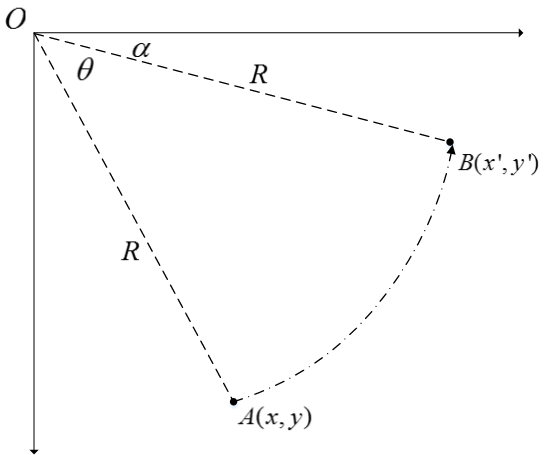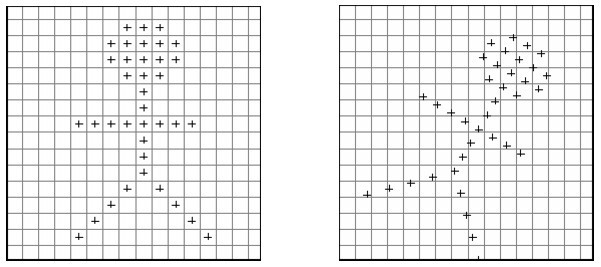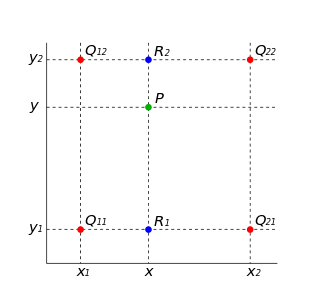# 仿射变换

• (x,y): 原图像中某一点A的位置
• (x,y): 变换后图像中A点对应的位置

## 几种常用的线性变换

### 平移（translation）

x=x+δxy=x+δy(1)

(xy)=(1001)(xy)+(δxδy)=(1001δxδy)xy1(2)

### 缩放（Scaling）

x=pxy=qy(3)

(xy)=(1001)(xy)+(δxδy)=(1001δxδy)xy1(4)

### 旋转（Rotation）x=Rcosαy=Rsinα(5)

A点可得：
x=Rcos(α+θ)y=Rsin(α+θ)(6)

x=xcosθ+ysinθy=xsinθ+ycosθ(7)

(xy)=(cosθsinθsinθcosθ)(xy)(8)

### 剪切(Shear)

(xy)=(1nm1)(xy)(9)

## 仿射变换

a=e=1,b=c=d=f=0，为恒等变换，即输入图像不变；当a=e=1,b=d=0时，为平移变换；当a=cosθ,b=sinθ,d=sinθ,e=cosθ,c=f=0时，为旋转变换；当a=e=1,c=f=0时，为剪切变换。当6个参数取其他值时，为一般的仿射变换，效果相当于从不同的位置看同一个目标。

# 双线性插值R1=x2xx2x1Q11+xx1x2x1Q21R2=x2xx2x1Q12+xx1x2x1Q22(11)

P=y2yy2y1R1+yy1y2y1R2(12)

P=(y2y)(x2x)(y2y1)(x2x1)Q11+(y2y)(xx1)(y2y1)(x2x1)Q21+(yy1)(x2x)(y2y1)(x2x1)Q12+(yy1)(xx1)(y2y1)(x2x1)Q22(13)

P=(y2y)(x2x)Q11+(y2y)(xx1)Q21+(yy1)(x2x)Q12+(yy1)(xx1)Q22(14)

# 参考文献©️2019 CSDN 皮肤主题: 编程工作室 设计师: CSDN官方博客